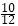# Compound Interest - Aptitude Questions and Answers

## Compound Interest Questions and Answers

Learn and practice the chapter "Compound Interest" with these solved Aptitude Questions and Answers. Each question in the topic is accompanied by a clear and easy explanation, diagrams, formulae, shortcuts and tricks that help in understanding the concept.

## Use of Compound Interest Questions

The questions and examples given in this section will be useful to all the freshers, college students and engineering students preparing for placement tests or any competitive exam like MBA, CAT, MAT, SNAP, MHCET, XAT, NMAT, GATE, Bank exams - IBPS, SBI, RBI, RRB, SSB, SSC, UPSC etc.
Practice with this online test to crack your placements and entrance tests!
1. Sum: 6400
Time period: 6 months
Rate of interest: 25% compounded quarterly.
Find the interest earned.

a. Rs. 735.5
b. Rs. 815
c. Rs. 825
d. Rs. 855

Explanation:

 Total Amount =  P1 + R2 100
Principal = P = Rs. 6400
 For quarterly compound interest, R = R and n = 4n 4
 Rate of interest, R = R = 25 4 4
 Time period, n = 4T = 4 x 6 months = 4 x 1 years = 2 years 2
 Total Amount =  64001 + 252 =  64004252 =  6400172 4 x 100 400 16
∴ Total Amount = Rs. 7225
Compound Interest = Amount - Principle = 7225 - 6400 = Rs. 825

Tip:
 For half yearly interest:- R = R and n = 2n 2
 For quarterly interest:- R = R and n = 4n 4
 For monthly interest:- R = R and n = 12n, 12
where n is in years.

2. Principal: 5000
Time: 46 months
Rate of interest : 30% pa.
Find the compound interest earned.

a. Rs. 8725.25
b. Rs. 8731.25
c. Rs. 8735.50
d. Rs. 8856.55

Explanation:

 Total Amount =  P1 + Rn 100
Principal = P = Rs. 5000
Rate of interest, R= 30% per annum
 if A completesTime period, n = 46 months = 3 years 10 months = 3 10 years 12

Tip:
 When time period is in fraction, say  n a years, use the below given formula - b
 Total Amount = P1 + Rn1 +R100 100

 Total Amount = 50001 + 3031 +x 30100 100
 Total Amount = 50001 + 331 + 110 4
 = 5000 x 13 x 13 x 13 x 5 10 10 10 4
∴ Total Amount = Rs. 13731.25
Compound Interest = Amount - Principle = 13731.25 - 5000 = Rs. 8731.25

3. Radhika wants to invest some amount for 3 years in a new scheme which says that the compound rate of interest for three years will be 5%, 12% and 8% respectively. How much investment will yield her Rs 6350.40 at the end of the investment period?

a. Rs. 5000
b. Rs. 5800
c. Rs. 6000
d. Rs. 6500

Explanation:

 Total Amount = Rs. 6350.40 = P1 + Rn 100
Rate of interest, R1 = 5% for 1st year; Time, n1 = 1 year (1st year)
R2 = 12% for 2nd year; n2 = 1 year (2nd year)
R3 = 8% for 3rd year; n3 = 1 year (3rd year)
 ∴ 6350.40 = P1 + 511 + 1211 + 81 100 100 100
 ∴ 6350.40 = P105112108100 100 100
 ∴ P = 6350.40 x 100 x 100 x 100 105 x 112 x 108
∴ P = Rs. 5000

4. Rohit says that, an amount became 4 times in 6 years. His friend wanted to know, how many years will it take for the amount to become 64 times, provided the rate of interest remains same?

a. 12 years
b. 16 years
c. 18 years
d. 24 years

Explanation:

Initial Amount = P.
P becomes 4 times in 6 years ∴ P →  4P in 6 years
∴ 4P → 4 x (4P) = 16P in next 6 years.
Continuing this, 16P → 4 x (16P) = 64P in next 6 years.
So total time to get to 64 times i.e. 64P = 6+6+6 = 18 years.

5. At the end of 3 years, the difference between the compound interest and simple interest comes to be Rs 320. The rate of interest is 25%. Find the principal amount.

a. Rs. 1525.50
b. Rs. 1545.78
c. Rs. 1550
d. Rs. 1575.38

Explanation:

Principal = P

 Compound Interest = Total Amount - Principal = P1 + Rn - P 100
 Simple Interest = PRT 100
R= 25% per annum; T and n = 3 years
Compound Interest - Simple Interest = Rs. 320
 P1 + Rn - P - PRT = 320 100 100
 ∴ P1 + 253 - P - P x 25 x 3 = 320 100 100
 ∴ P53 - P - 3P = 320 4 4
 ∴ P125-P + 3P= 320 64 4
 ∴ 125P - 7P = 320 64 4
∴ P = Rs. 1575.38